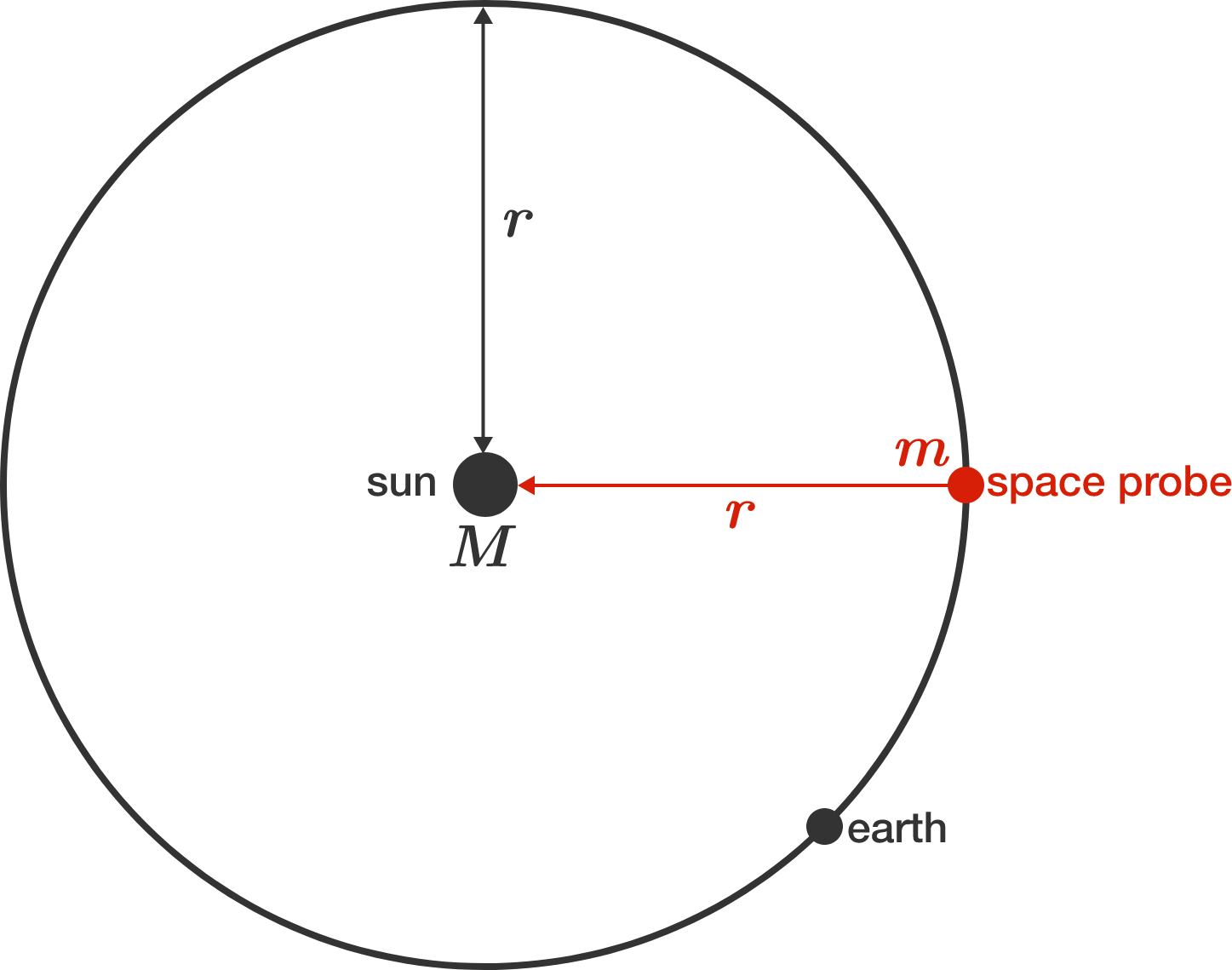# Fall into the sun

A space probe is on a circular orbit around the sun at a distance of $r_0 = 1 \,\text{AU}$ (astronomical unit). It collides with an asteroid in its trajectory, losing all of its kinetic energy. As a result, the space probe falls directly towards the sun.

How long (in days) does it take for the space probe to arrive at the sun? Round to the nearest integer.Details and Assumptions: The Earth orbits the sun in an elliptical orbit with semi-major axis $1 \text{ AU},$ and has an orbital period of 365.25 days. The planets (including the Earth) have a negligible impact on the orbit of the probe. The diameter of the sun is negligible compared to the orbital radius $r_0.$

×